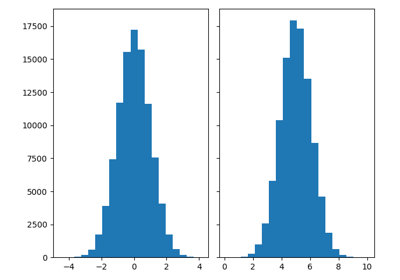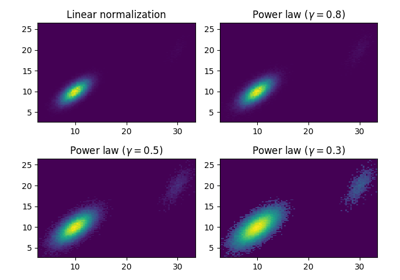You are reading an old version of the documentation (v3.1.3). For the latest version see https://matplotlib.org/stable/api/_as_gen/matplotlib.axes.Axes.hist2d.html

# matplotlib.axes.Axes.hist2d¶

Axes.hist2d(self, x, y, bins=10, range=None, density=False, weights=None, cmin=None, cmax=None, *, data=None, **kwargs)[source]

Make a 2D histogram plot.

Parameters: x, y : array_like, shape (n, ) Input values bins : None or int or [int, int] or array_like or [array, array] The bin specification: If int, the number of bins for the two dimensions (nx=ny=bins). If [int, int], the number of bins in each dimension (nx, ny = bins). If array_like, the bin edges for the two dimensions (x_edges=y_edges=bins). If [array, array], the bin edges in each dimension (x_edges, y_edges = bins). The default value is 10. range : array_like shape(2, 2), optional, default: None The leftmost and rightmost edges of the bins along each dimension (if not specified explicitly in the bins parameters): [[xmin, xmax], [ymin, ymax]]. All values outside of this range will be considered outliers and not tallied in the histogram. density : bool, optional, default: False Normalize histogram. normed is a deprecated synonym for this parameter. weights : array_like, shape (n, ), optional, default: None An array of values w_i weighing each sample (x_i, y_i). cmin : scalar, optional, default: None All bins that has count less than cmin will not be displayed and these count values in the return value count histogram will also be set to nan upon return cmax : scalar, optional, default: None All bins that has count more than cmax will not be displayed (set to none before passing to imshow) and these count values in the return value count histogram will also be set to nan upon return h : 2D array The bi-dimensional histogram of samples x and y. Values in x are histogrammed along the first dimension and values in y are histogrammed along the second dimension. xedges : 1D array The bin edges along the x axis. yedges : 1D array The bin edges along the y axis. image : QuadMesh cmap : Colormap or str, optional A colors.Colormap instance. If not set, use rc settings. norm : Normalize, optional A colors.Normalize instance is used to scale luminance data to [0, 1]. If not set, defaults to colors.Normalize(). vmin/vmax : None or scalar, optional Arguments passed to the Normalize instance. alpha : 0 <= scalar <= 1 or None, optional The alpha blending value.

See also

hist
1D histogram plotting

Notes

• Currently hist2d calculates it's own axis limits, and any limits previously set are ignored.
• Rendering the histogram with a logarithmic color scale is accomplished by passing a colors.LogNorm instance to the norm keyword argument. Likewise, power-law normalization (similar in effect to gamma correction) can be accomplished with colors.PowerNorm.

Note

In addition to the above described arguments, this function can take a data keyword argument. If such a data argument is given, the following arguments are replaced by data[<arg>]:

• All arguments with the following names: 'weights', 'x', 'y'.

Objects passed as data must support item access (data[<arg>]) and membership test (<arg> in data).

## Examples using matplotlib.axes.Axes.hist2d¶HistogramsExploring normalizations